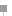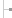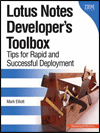Get started Bring yourself up to speed with our introductory content.

# What is Lotus Formula language?

## Learn the basics of Formula language for Lotus Notes

The following is tip #1 from "A Formula language for Lotus Notes introduction -- 7 tips in 7 minutes," excerpted from Chapter 5 of the book Lotus Notes Developer's Toolbox , published by IBM Publishing.

What is Formula language?

Formula language is a simple, easy-to-use programming language that can be found in many Lotus products -- such as Lotus 1-2-3 and Lotus Notes. Formula language has been integrated into Lotus Notes since its inception in 1989 and has included numerous enhancements over the years.

Then, in 2002, Lotus decided to completely rewrite the underlying processor (or engine) used to interpret the formula language source code. This significantly improved its performance and expanded its capabilities. Using a few simple Formula language commands and functions, you can build a robust database application.

What is a Formula?

In the simplest terms, formulas are used to produce a data value or action. This value or action is derived by using one or more commands, functions, or statements to create a main expression. All formulas must have a main expression that equates to a result. If the formula does not produce a value or action, the Designer client will not allow you to save the formula.

For example, the following illustrates an invalid formula. Here, the text value "Happy New Year" is assigned to a temporary variable called message. Although the formula does perform a calculation, there is no main expression. This formula does not produce a result.

```message := "Happy New Year";
```

For this example to be valid, the formula must do something to produce a result. This second example illustrates a valid formula. Looking at this formula, you'll notice that the first line performs a computation by assigning a text value to a temporary variable. The statement is terminated with a semicolon, which separates the first statement from the second. The formula then ends with the main expression, which defines the value for the field.

```message := "Happy New Year";
message
```

In this case, the main expression is assigned the contents of the variable message. Alternatively, the same result could have been achieved by setting the text string to be the main expression, as illustrated next.

```"Happy New Year"
```

Tip: When writing a formula containing multiple statements, each statement must be separated by a semicolon, and the last line in the formula must be the "main expression."A Formula language for Lotus Notes introductionHome: IntroductionTip 1: What is Lotus Formula Language?Tip 2: Working with variables in Formula language for Lotus NotesTip 3: Lotus Notes Formula language keywordsTip 4: Working with operators in Formula language for Lotus NotesTip 5: Lotus Notes Formula language general syntax rulesTip 6: What are Lotus Notes Formula language functions and commands?Tip 7: Working with text strings in Formula language for Lotus NotesThis chapter excerpt from Lotus Notes Developer's Toolbox, by Mark , is printed with permission from IBM Publishing, Copyright 2006. Click here for the chapter download or purchase the book here.

#### Dig Deeper on Lotus Notes 5

• Favorite iSeries cheat sheets

Here you'll find a collection of valuable cheat sheets gathered from across the iSeries/Search400.com community. These cheat ...

Close